# 如何用 canvas 渲染 Web Excel 富文本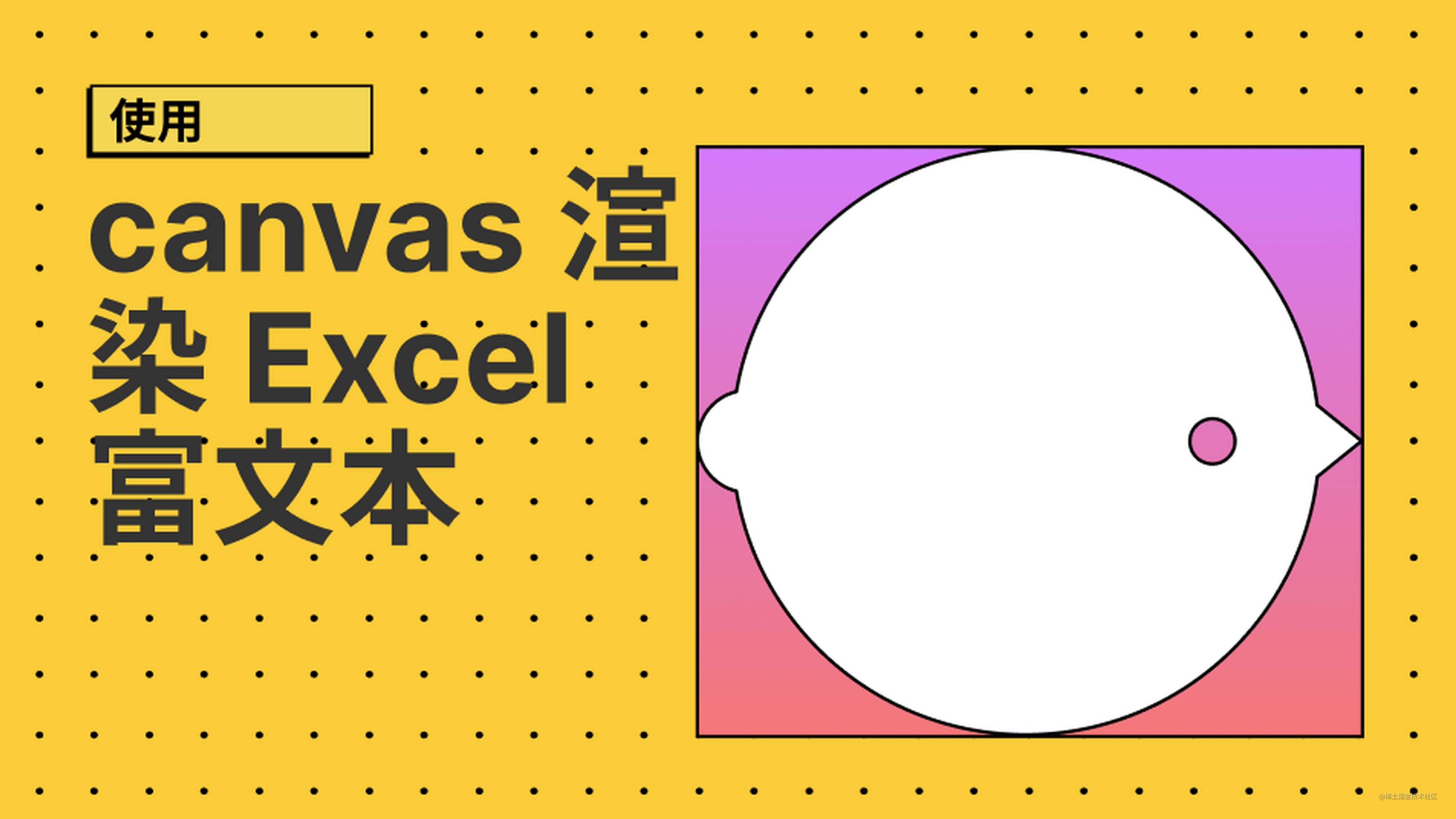## 自动换行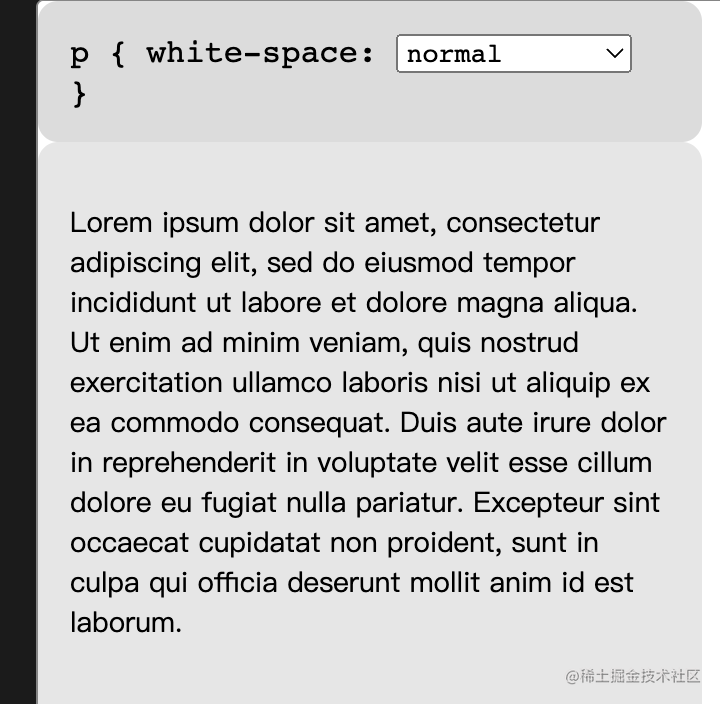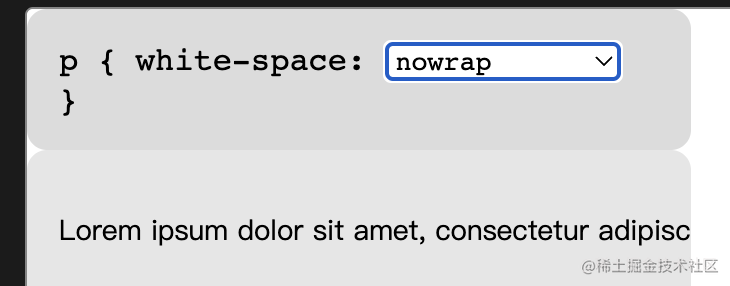canvas 中的 `measureText` API 可以用来测量文本的信息，它返回一个 TextMetrics 对象，签名如下所示。

``````interface TextMetrics {
// x-direction
readonly attribute double width; // advance width
readonly attribute double actualBoundingBoxLeft;
readonly attribute double actualBoundingBoxRight;

// y-direction
readonly attribute double fontBoundingBoxAscent;
readonly attribute double fontBoundingBoxDescent;
readonly attribute double actualBoundingBoxAscent;
readonly attribute double actualBoundingBoxDescent;
readonly attribute double emHeightAscent;
readonly attribute double emHeightDescent;
readonly attribute double hangingBaseline;
readonly attribute double alphabeticBaseline;
readonly attribute double ideographicBaseline;
};

TextMetrics 中的 `width` 表示当前测量字符的宽度，`fontBoundingBoxAscent``fontBoundingBoxDescent` 可以知道这一行的高度。

``````const text = 'abcdefg'

let maxWidth = 100
let lineWidth = 0
let w = 0
let line = ''
for (let c of text) {
w = ctx.measureText(c).width
if (totalWidth + w > maxWidth) {
console.log(line)
line = c
lineWidth = w
} else {
line += c
lineWidth += w
}
}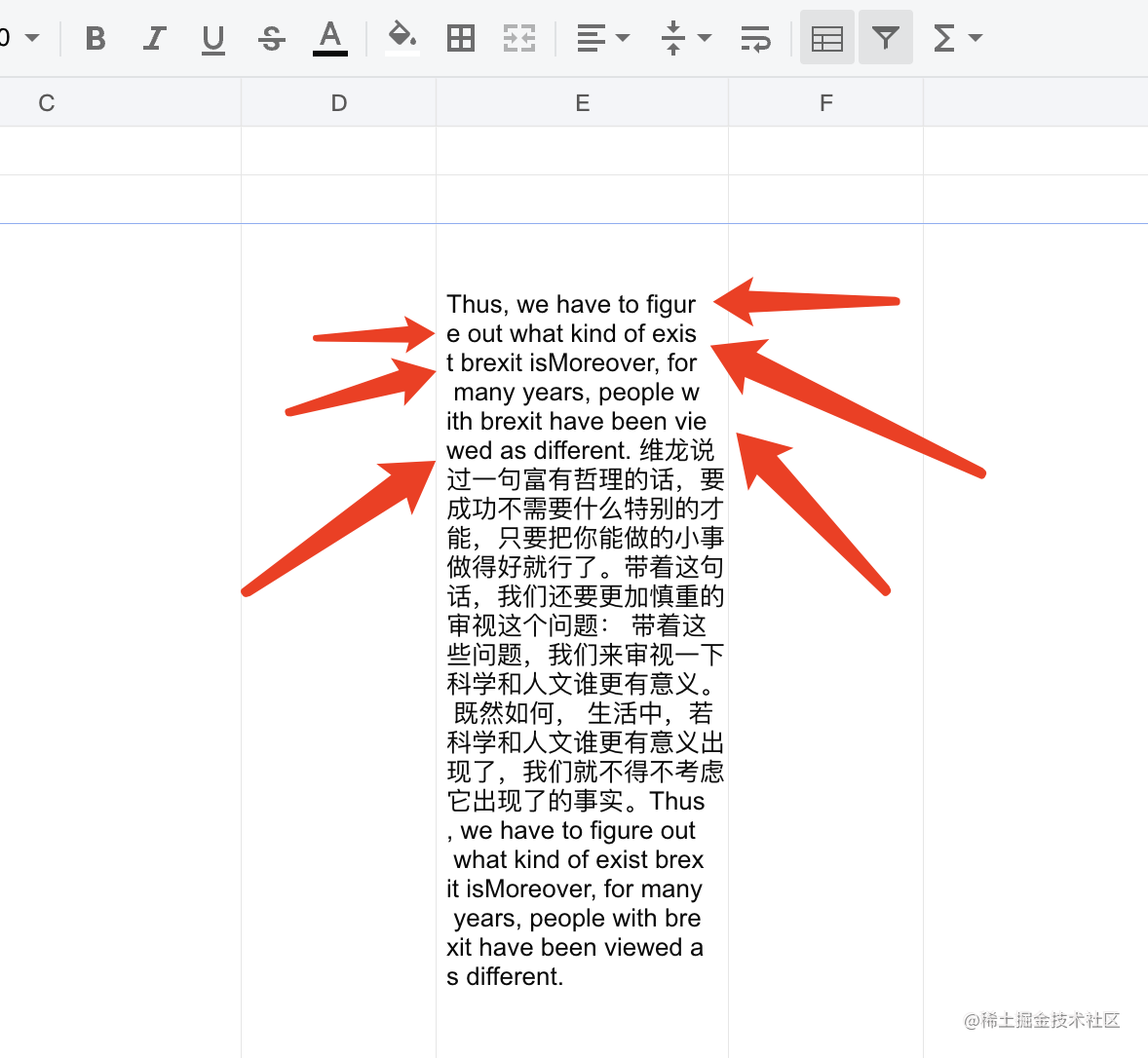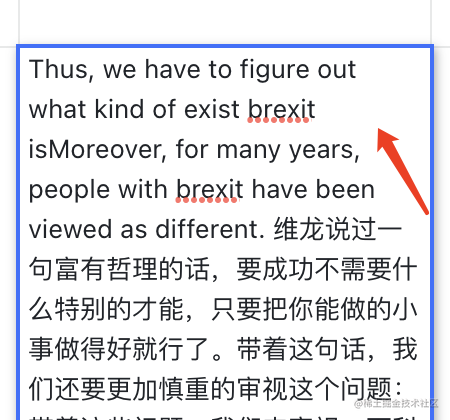``````const breakCharSet = new Set(['?', '-', ' ', ',', '.'])
function isWordBreakChar(ch) {
if (ch.charCodeAt(0) < 0x2e80) return breakCharSet.has(ch)
return true
}

``````const lines = []
let line = ''
let word = ''
let lineWidth = 0
let wordWidth = 0
for (let c of text) {
const w = ctx.measureText(c)
const inWord = !isWordBreakChar(c)

if (lineWidth + wordWidth + w > maxWidth) { // 如果超长
if (lineWidth) {
lines.push(line)
line = ''
lineWidth = 0

if (wordWidth + w > maxWidth) {
if (wordWidth) {
lines.push(word)
word = c
wordWidth = w
}
if (w > maxWidth) {
lines.push(c)
word = ''
wordWidth = 0
}
} else if (!inWord) {
line += (word + c)
lineWidth += (wordWidth + w)
word = ''
wordWidth = 0
} else {
word += ch
wordWidth += w
}

} else if (wordWidth) {
lines.push(word)
word = c
wordWidth = w
} else { // 如果容器宽度小于一个字符
lines.push(c)
}
} else if (inWord) { // 如果属于一个单词
word += ch
wordWidth += w
} else { // 如果不是一个单词
line += (word + c)
lineWidth += (wordWidth + w)
word = ''
wordWidth = 0
}

}

## 富文本

``````interface Rich {
start: number; // 开始字符（包含）
end: number; // 结束字符（不包含）

fontFamily?: string; // 字体
fontSize?: number; // 字体大小
bold?: boolean; // 是否加粗
italic?: boolean; // 是否倾斜

color?: string; // 颜色

underline?: boolean; // 下划线
lineThough?: boolean; // 删除线
}

`Rich` 接口定义了原文本 `start``end` 范围内的样式，这里一共定义了 7 种富文本样式，前 4 个可以用 canvas 中的 `font` 来实现，颜色可以用 `fillStyle`，而下划线和删除线则需要我们自己来实现，在特定位置画一条横线。

``````interface TextData {
width: number; // 容器宽度
text: string; // 要渲染的文本
rich?: Rich[] // 当前文本的富文本样式
}

``````function getFont(r) {
return `\${r.italic ? 'italic' : ''} \${r.bold ? 'bold' : ''} \${r.fontSize || 16}px \${r.fontFamily || 'sans-serif'}`.trim()
}

function measureText(str, font) {
ctx.font = font
return ctx.measureText(str)
}

`TextCell` 是文本容器，它拥有多个 `TextLine``TextLine` 是一个行文本，它包含多个 `TextToken``TextToken` 是是个文本片段，这一个文本片段的样式要是一样的（属于同一个 Rich）。

``````let prevEnd = 0
for (let i = 0, r; i < richLen; ++i) {
r = rich[i] // 富文本配置
if (prevEnd < r.start) {
// 纯文本
flush(parseText(text.slice(prevEnd, r.start), x, maxWidth))
}

// 富文本
flush(parseText(text.slice(r.start, r.end), x, maxWidth, r))
prevEnd = r.end
}

`flush` 是创建 `TextLine` 如果当前文本长度超了的话，另外它还会修改 `TextToken` 的高度，比如先解析字体比较小的 `TextToken`，如果后面又遇到这一行中字号更大的 `TextToken` 则需要手动修改之前 `TextToken` 的高度。

``````let prevEnd = 0
let x = 0
let j = 0
let len = 0
let line = []
let lineHeights = []
const lines = []

const flushLine = () => {
lines.push(new TextLine(line, Math.max.apply(null, lineHeights))) // 修改行高
}
const flush = (info) => {
j = 0
while (info.tokens[j]?.x) j++
len = info.tokens.length
if (j < len) {
if (line.length) { // 说明当前 TextToken 超了一行
line.push(...info.tokens.slice(0, j))
if (j) lineHeights.push(info.lineHeight)
flushLine() // 完成一行
line = []
lineHeights = []
}

if ((len - j - 1) > 0) {
for (let l = len - 1; j < l; ++j) { // 每一个 TextToken 就是一行
lines.push(new TextLine([info.tokens[j]], info.lineHeight))
}
}

line.push(info.tokens[len - 1]) // 保留最后一个
} else {
line.push(...info.tokens)
}

lineHeights.push(info.lineHeight)
x = info.x // 一下个代解析片段的起始 x
}

## 渲染

``````function render(cellData) {
const cell = new TextCell(cellData)
ctx.save();

ctx.strokeRect(0, 0, cell.width, cell.height);

ctx.beginPath();
ctx.rect(0, 0, cell.width, cell.height);
ctx.clip();

let dx = 4 // padding
let dy = 0

cell.lines.forEach(l => {
l.tokens.forEach(t => {
ctx.font = t.style.font
ctx.strokeStyle = ctx.fillStyle = t.style.color || '#000'
ctx.fillText(t.text, t.x + dx, l.y + dy) // 渲染文字

if (t.style.underline) { // 渲染下划线
ctx.beginPath();
ctx.moveTo(t.x + dx, l.y+3 + dy);
ctx.lineTo(t.x + t.width + dx, l.y+3 + dy);
ctx.stroke();
}
if (t.style.lineThough) { // 渲染删除线
ctx.beginPath();
ctx.moveTo(t.x + dx, l.y - t.actualHeight / 2 + dy);
ctx.lineTo(t.x + t.width + dx, l.y - t.actualHeight / 2 + dy);
ctx.stroke();
}
})
})

ctx.restore();
}